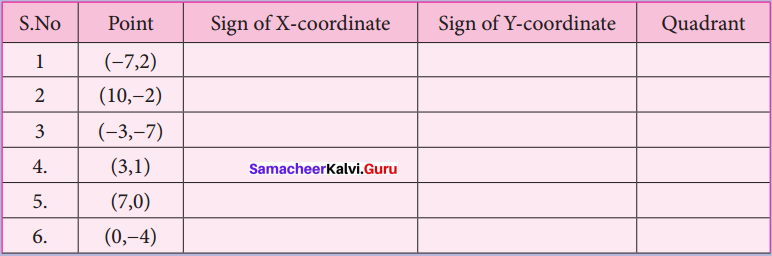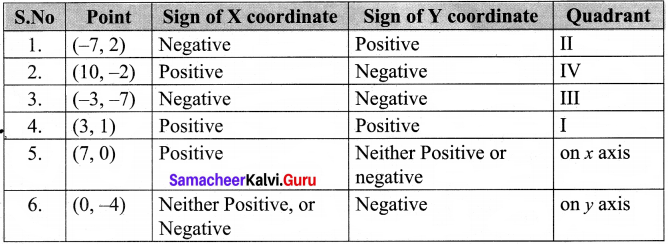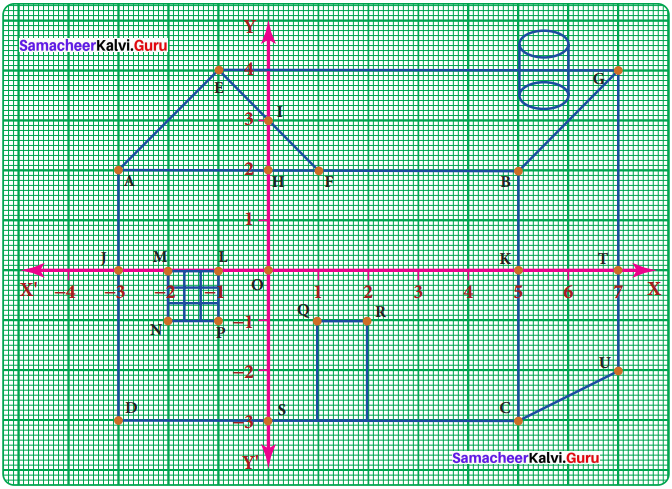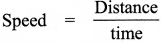# Samacheer Kalvi 8th Maths Solutions Term 2 Chapter 2 Algebra Intext Questions

Students can Download Maths Chapter 2 Algebra Intext Questions and Answers, Notes Pdf, Samacheer Kalvi 8th Maths Book Solutions Guide Pdf helps you to revise the complete Tamilnadu State Board New Syllabus and score more marks in your examinations.

## Tamilnadu Samacheer Kalvi 8th Maths Solutions Term 2 Chapter 2 Algebra Intext Questions

Exercise 2.1
Try These (Text book page no. 32)

Question 1.
Identify which among the following are linear equations.

1. 2 + x = 19 – Linear as degree of the variable x is 1
2. 7x2 – 5 = 3 – not linear as highest degree of x is 2
3. 4p3 = 12 – not linear as highest degree ofp is 3
4. 6m + 2 – Linear, but not an equation
5. n = 10 – Linear equation as degree of n is 1
6. 7k – 12= 0 – Linear equation as degree of Hs 1
7. $$\frac{6x}{8}$$ + y = 1 – Linear equation as degree ofx & y is 1
8. 5 + y = 3x – Linear equation as degree ofy & x is 1
9. 10p + 2q = 3 – Linear equation a& degree ofp & q is 1
10. x2 – 2x-4 – not linear equation as highest degree of x is 2Think (Text book page no. 32)

Question 1.
Is t(t – 5)= =10 a linear equation? Why?
Solution:
t(t – 5) = 10
= t x t – 5 x t = 10
= t2 – 5t = 10
This is not a linear equation as the highest degree of the variable ‘t’ is 2

Question 2.
Is x2 = 2x, a linear equation? Why?
Solution:
x2 = 2x
= x2 – 2x = 0
This is not a linear equations as the highest degree of the variable ‘x’ is 2

Try These (Text book page no. 33)

Convert the following statements into linear equations:

Question 1.
On subtracting 8 from the product of 5 and a number, I get 32.
Solution:
Convert to linear equations:
Given that on subtracting 8 from product of 5 and a, we get 32
5 × x – 8 = 32
5x – 8 = 32Question 2.
The sum of three consecutive integers is 78.
Solution:
Sum of 3 consecutive integers is 78
Let 1st integer be ‘x’
∴ x + (x + 1) + (x + 2) = 78
∴ x + x + 1 + x + 2 = 78
3x + 3 = 78

Question 3.
Peter had a Two hundred rupee note. After buying 7 copies of a book he was left with ₹ 60.
Solution:
Let cost of one book be ‘x’
∴ Given that 200 – 7 × x = 60
∴ 200 – 7x = 60

Question 4.
The base angles of an isosceles triangle are equal and the vertex angle measures 80°.
Solution:
Let base angles each be equal to x & vertex bottom angle is 80°. Applying triangle
property, sum of all angles is 180°
∴ x + x + 80 = 180°
2x + 80 = 180°Question 5.
In a triangle ABC, ∠A is 10° more than ∠B. Also ∠C is three times ∠A. Express the equation in terms of angle B
Solution:
Let ∠B = b
Given ∠A = 10° + ∠B = 10 + b
Also given that ∠C = 3 x ∠A = 3 x (10 + 6) = 30 + 3b
Sum of the angles = 180°
∠A + ∠B + ∠C = 180°
10 + b + b + 30 + 3b = 180°
∴ 5b + 40 = 180°

Think (Text book page no. 34)

Question 1.
Can you get more than one solution for a linear equation?
Solution:
Yes, we can get. Consider the below line or equation
x + y = 5
here, when x = 1, y = 4
when x = 2, y = 2
x = 3, y = 2
x = 4, y = 1
Hence, we get multiple solutions for the same linear equation.

Think (Text book page no. 35)

Question 1.
“An equation is multiplied or divided by a non zero number on either side.” Will there be any change in the solution?
Solution:
Not be any change in the solutionQuestion 2.
“An equation is multiplied or divided by two different numbers on either side”. What will happen to the equation?
Solution:
When an equation is multiplied or divided by 2 different numbers on either side, there will be a change in the equation & accordingly, solution will also change.

Exercise 2.2
Think (Text book page no. 37)

Question 1.
Suppose we take the second piece to be x and the first piece to be (200 – x), how will the steps vary. Will the answer be different?
Solution:
Let 2nd piece be V & 1st piece is 200 – x
Given that 1st piece is 40 cm smaller than hence the other piece
∴ 200 – x = 2 × x – 40200 + 40 = 2x + x
240 = 3x
∴ x = $$\frac{240}{3}$$ = 80
∴ 1st piece = 200 – x = 200 – 80 = 120 cm
2nd piece = x = 80 cm

Exercise 2.3
Think (Text book page no. 43)

Question 1.
If instead of (4, 3), we write (3, 4) and try to mark it, will it represent ‘M’ again?
Solution:
Let 3, 4 be M, when we mark, we find that it is a different point and not ‘M’

Try These (Text book page no. 45)

Question 1.
Complete the table given below.Solution:Question 2.
Write the coordinates of the points marked in the following figure• A – (-3, 2)
• B – (5, 2)
• C – (5, -3)
• D – (-3, 3)
• E – (-1,4)
• F – (1, 2)
• G – (7, 4)
• H – (0, 2)
• i – (o, 3)
• J – (-3, 0)
• K – (5, 0)
• L – (-1, 0)
• M – (-2, 0)
• N – (-2, -1)
• O – (0, 0)
• P – (-1, -1)
• Q – (1, -1)
• R – (2, -1)
• S – (0, -3)
• T – (7, 0)
• U – (7, -2)

Exercise 2.4
Think (Text book page no. 49)

Question 1.
Which of the points (5, -10) (0, 5) (5, 20) lie on the straight line X = 5?
Solution:
All points on the line X = 5 will have X – coordinate as 5.
Therefore, any point with X – coordinate as 5 will lie on X = 5 line.
Hence the points (5, – 10) & (5,20) will lie on X = 5

Think (Text book page no. 54)Question 1.
Why is it given that the speed is ‘constant’? If the speed is not constant, will the graph be the same? The graph is named as y = 80 x algebraically. Why?
Solution:or in other words, the slope of a line in a distance – time graph. We observe that the slope of the graph is constant hence, speed is constant. If the speed is not constant, the graph will be different as slope of the line would change.